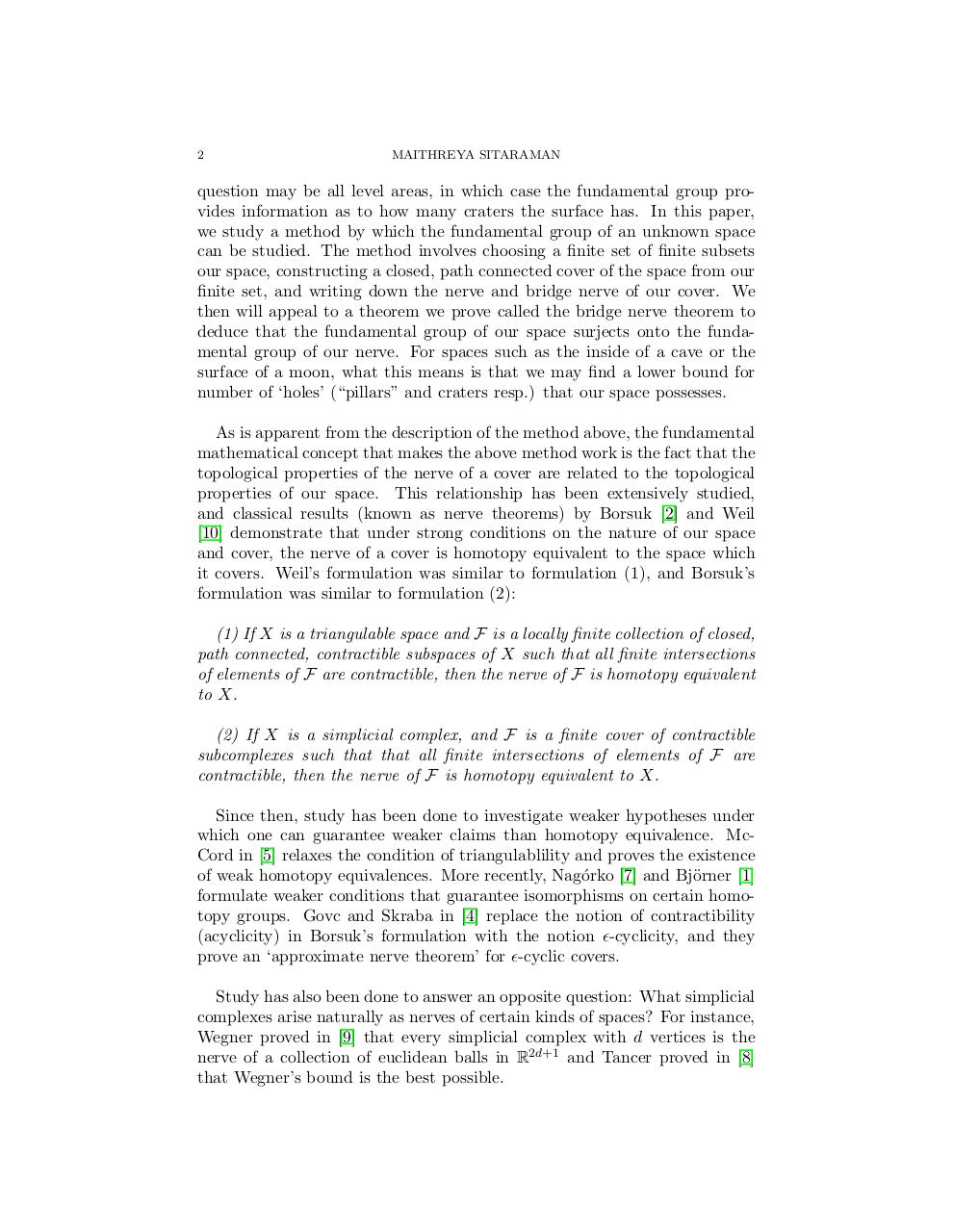# Exploring spaces Oct 10.4.pdfPage 1 23424

#### Text preview

2

MAITHREYA SITARAMAN

question may be all level areas, in which case the fundamental group provides information as to how many craters the surface has. In this paper,
we study a method by which the fundamental group of an unknown space
can be studied. The method involves choosing a finite set of finite subsets
our space, constructing a closed, path connected cover of the space from our
finite set, and writing down the nerve and bridge nerve of our cover. We
then will appeal to a theorem we prove called the bridge nerve theorem to
deduce that the fundamental group of our space surjects onto the fundamental group of our nerve. For spaces such as the inside of a cave or the
surface of a moon, what this means is that we may find a lower bound for
number of ‘holes’ (“pillars” and craters resp.) that our space possesses.
As is apparent from the description of the method above, the fundamental
mathematical concept that makes the above method work is the fact that the
topological properties of the nerve of a cover are related to the topological
properties of our space. This relationship has been extensively studied,
and classical results (known as nerve theorems) by Borsuk  and Weil
 demonstrate that under strong conditions on the nature of our space
and cover, the nerve of a cover is homotopy equivalent to the space which
it covers. Weil’s formulation was similar to formulation (1), and Borsuk’s
formulation was similar to formulation (2):
(1) If X is a triangulable space and F is a locally finite collection of closed,
path connected, contractible subspaces of X such that all finite intersections
of elements of F are contractible, then the nerve of F is homotopy equivalent
to X.
(2) If X is a simplicial complex, and F is a finite cover of contractible
subcomplexes such that that all finite intersections of elements of F are
contractible, then the nerve of F is homotopy equivalent to X.
Since then, study has been done to investigate weaker hypotheses under
which one can guarantee weaker claims than homotopy equivalence. McCord in  relaxes the condition of triangulablility and proves the existence
of weak homotopy equivalences. More recently, Nag´orko  and Bj¨orner 
formulate weaker conditions that guarantee isomorphisms on certain homotopy groups. Govc and Skraba in  replace the notion of contractibility
(acyclicity) in Borsuk’s formulation with the notion -cyclicity, and they
prove an ‘approximate nerve theorem’ for -cyclic covers.
Study has also been done to answer an opposite question: What simplicial
complexes arise naturally as nerves of certain kinds of spaces? For instance,
Wegner proved in  that every simplicial complex with d vertices is the
nerve of a collection of euclidean balls in R2d+1 and Tancer proved in 
that Wegner’s bound is the best possible.